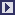## PCPACK6 Equation Editor Tool

 The Equation Editor is available as part of the PCPACK suite of tools. It is used to create and edit equations for use in annotation pages created by the Annotation Tool. The Equation Editor allows you to create all types of mathematical and logical expressions. It also allows multiple equations to be created so that calculations can be shown. The equations that you create in the Equation Editor are saved in a format called MathML which is the latest means of displaying equations on internet sites (i.e. what HTML is to text, MathML is to mathematical expressions). Hence, the Equation Editor provides a universal way of creating equations for use on web pages (and can be used as a standalone tool for this purpose).

Other PCPACK tools:Protocol ToolLadder ToolDiagram ToolMatrix ToolAnnotation ToolAdmin ToolPublisher ToolDiagram Template ToolLauncher Wizard Tool